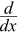# Power Rule

The Power Rule, one of the most commonly used rules in Calculus, says:

The derivative of   xn   is   nx(n-1)

### Example: What is the derivative of x2 ?

For x2 we use the Power Rule with n=2:

 The derivative of   x2 = 2x(2-1) = 2x1 = 2x

Answer: the derivative of x2 is 2x

"The derivative of" can be shown with the little mark

So we get this definition:

f’(xn) = nx(n-1)

### Example: What is the derivative of x3 ?

f’(x3) = 3x3−1 = 3x2

"The derivative of" can also be written d dx

### Example: What is(1/x) ?

1/x is also x-1

Using the Power Rule with n = −1:xn = nxn−1x−1 = −1x−1−1 = −x−2

## How to Remember"multiply by power
then reduce power by 1"

## A Short Table

Here is the Power Rule with some sample values. See the pattern?

f f’(xn) = nx(n-1) f’
x 1x(1-1) = x0 1
x2 2x(2-1) = 2x1 2x
x3 3x(3-1) = 3x2 3x2
x4 4x(4-1) = 4x3 4x3
etc...

And for negative exponents:
x-1 -1x(-1-1) = -x-2 -x-2
x-2 -2x(-2-1) = -2x-3 -2x-3
x-3 -3x(-3-1) = -3x-4 -3x-4
etc...